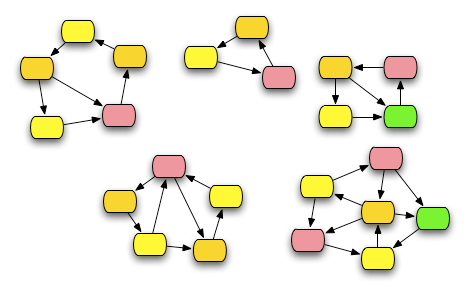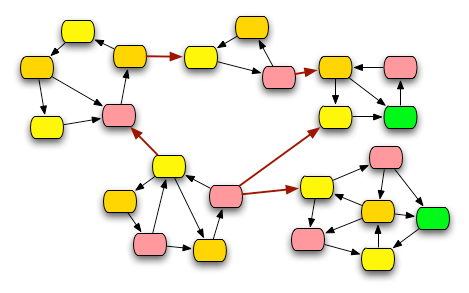# Making Graph Algorithms Fast, using Strongly Connected Components

One of the problems with many of the interesting graph algorithms is that they’re complex. As graphs get large, computations over them can become extremely slow. For example, graph coloring is NP-complete – so the time to run a true optimal graph coloring algorithm on an arbitrary graph grows exponentially in the size of the graph!

So, quite naturally, we look for ways to make it faster. We’ve already talked about using
heuristics to get fast approximations. But what if we really need the true optimum? The other approach to making it faster, when you really want the true optimum, is parallelism: finding some way to subdivide
the problem into smaller pieces which can be executed at the same time. This is obviously a limited
technique – you can’t stop the exponential growth in execution time by adding a specific finite
number of processors. But for particular problems, parallelism can make the difference between
being able to do something fast enough to be useful, and not being able to. For a non-graph
theoretic but compelling example, there’s been work in something called microforecasting, which is precise weather forecasting for extreme small areas. It’s useful for predicting things like severe lightning storms in local areas during events where there are large numbers of people. (For example, it was used at the Atlanta olympics.) Microforecasting inputs a huge amount of information about local conditions – temperature, air pressure, wind direction, wind velocity, humidity, and such, and computes a short-term forecast for that area. Microforecasting is completely useless if it takes longer to compute the forecast than it takes for the actual weather to develop. If you can find a way to split the computation among a hundred processors, and get a forecast in 5 minutes, you’ve got something really useful. If you have to run it on a single processor, and it takes 2 hours – well, by then, any storm
that the forecast predicts is already over.

For graphs, there’s a very natural way of decomposing problems for parallelization. Many complex graphs for real problems can be divided into a set of subgraphs, which can be handled in parallel. In
fact, this can happen on multiple levels: graphs can be divided into subgraphs, which can be divided
into further subgraphs. But for now, we’ll mainly focus on doing it once – one decomposition of
the graph into subgraphs for parallelization.

For any graph, directed or undirected, we have a notion of connectedness. A graph is
connected if, for any two vertices A and B in the graph, there’s a path between them. For directed
graphs, we can define a closely related concept: strong connection. Two vertices v1 and v2 in a digraph are connected if/f there’s a path between them – that is,
if there’s a path from v1 to v2, and there’s also a path
from v2 to v1. The digraph is strongly connected if and only if every pair of vertices in it are strongly connected.

Now, suppose we have a digraph which is connected, but not strongly connected. Then
one of the things we can do with that graph is look at ways of partitioning the graph into
strongly-connected subgraphs. There are a bunch of reasons why doing that it useful: for example, there are many algorithms that can be implemented in a divide-and-conquer fashion by identifying the
strongly connected components of the graph, performing the algorithm on those components, and then merging the results.

The way that works is pretty simple. You find the strongly connected components, and contract each of
them to a single vertex. You end up with a new graph. You can compute properties of the graph by
dividing them into the strongly connected components – computing the things for each of the components, and them merging the results by using the contracted graph. If the graph is large enough, you can divide that contracted graph into its own strongly connected subgraphs.

For example, you can use this for graph coloring. Find the strongly connected components – and color those. Then you can merge the coloring results by doing a join of the strongly connected components, adding colors when necessary at the joints.

For example, look at the graph below. We can divide it into its strongly connected components, which I’ve marked off by dotted lines.Now, we can color each of the strongly connected components separately, producing the graph below.Then we go back to the full graph – but we treat only look at the edges that join
the strongly connected components. And we re-shuffle colors to avoid conflicts where we’re joining subgraphs. When we can’t avoid a conflict by re-shuffling, we would add a color – but that isn’t necessary in this case. The end-result is shown below.Doing it this way has two advantages. One is the fact that it’s so easily parallelizable: each of the strongly connected components can be colored simultaneously, and then
the results merged. In terms of the common current models of parallel programming, this – like many other graph algorithms built using subdivision to strongly connected components – is a perfect candidate for implementation as a map-reduce.

The other advantage is that it’s actually a potential complexity reducer. That is,
doing this sequentially, finding a coloring for the strongly connected components and then merging, is an application of a useful heuristic that can, in general, speed up
the graph coloring. In some cases, the merging of component colorings can be expensive enough to outweigh the savings of doing a group of smaller colorings, but
in general, for most graphs that appear in many real problems, the component coloring and merge is a faster way of finding the optimal coloring.

Next post, I’ll talk about algorithms for finding the strongly connected components: after all, using SCCs as a tool in this way only makes sense if we can find the SCCs quickly!

## 0 thoughts on “Making Graph Algorithms Fast, using Strongly Connected Components”

1.Kathryn Cramer

Dear Mark:
I don’t see an email address for you, so I’m writing via your comment section.
I thought you be interested to know who won Stephen Wolfram’s 2,3 Turing machine contest, proving that the 2,3 Turing machine is universal: a 20-year old engineering student named Alex Smith.
The write-up in Nature is here:
http://www.nature.com/news/2007/071024/full/news.2007.190.html
and Stephen Wolfram’s blog entry on it is here:
http://blog.wolfram.com/2007/10/the_prize_is_won_the_simplest.html?lid=title
The New Scientist story, that has good biographical detail is here:
http://technology.newscientist.com/article/dn12826-simplest-universal-computer-wins-student-25000.html
Smith’s 44 page proof is here: http://www.wolframscience.com/prizes/tm23/TM23Proof.pdf

2.ukko

Just an aside, are you using OminGraffle for your graphs?

3.Mark C. Chu-Carroll

ukko:
Yes, I use omnigraffle for pretty much all of the diagrams that I draw. I should probably just put a footer on my posts saying “diagrams by omnigraffle”.

4.Jason Adams

Looking forward to the next post on finding SCCs. Stuff like this is why I love this blog.

5.Coin

This is very interesting.
I’d be curious whether this could plausibly be used as a way of [I’m not sure what the best terminology for what I’m trying to describe is?] reducing amortized complexity– i.e. you have a graph which you need to do several calculations on, you calculate the strongly connected components once and then each of your successive graph operations get a speed boost.
What is the complexity to find the strongly connected components? (Or should I just wait until the next post for that one…)

6.Josef Svenningsson

While strongly connected components are a nice tool for speeding up certain algorithms I think it’s important to point out that many graph algorithms are inherently non-parallelizable, being p-time complete. One such algorithm is the transitive closure. Any attempt at parallelize such algorithms will most certainly make them slower.

7.Doug

I do find this interesting, but any more DETAILED examples? You mentioned weahter. But, what information do we shuffle around? What do we have for each node of a sample strongly connected sub-network? I mean I can see that we can’t have matching colors up there, so we do a shuffle within a strongle connected network. However, I’m sure this applies to more than just mere color matching. I can’t say I can think of an example where one needs to do this or how this helps to split computations among computers (obviously I don’t know much about computers in this respect, so please englihten me).

8.Vlad Shcherbina

A contracted graph is a DAG, so it’s impossible to perform further subdivision.

9.Alex Rubinsteyn

Thanks for posting this article, it’s very readable and the diagrams helped me digest it even faster. I’m curious is the graph coloring you get with the above method optimal? If not, do you know any bounds on how many extra colors this algorithm will use relative to a graph’s chromatic number?

10.Josh

Mark,
Thanks for blogging on this. I must admit that I haven’t found time to read most of your recent posts (that’s thanks to taking four CSCI classes plus a physics class), but I know I’ll go back to read them when things are less hectic.
This one is timely for me, too, since one of my classes has been covering various graph algorithms over the last few weeks. I’m interested to see how you describe the SCC algorithm.

11.I. Cahit

The idea of “first find strongly connected components and then color in parallel” in order speeding up efficiency of the coloring algorithm has been used in graph coloring problems of planar graphs in a different way. That is “first find spiral chains and then color each spiral chain one by one”. This type methods may also give short algorithmic proofs of the some graph colorings conjectures/theorems as well.
See my recent paper “A Unified Spiral Chain Coloring Algorithm for Planar Graphs” (http://arxiv.org/abs/0710.2066).

12.Martin

These graphs look really nice! What software are you using for drawing them? I doubt that Graphviz can do that.

13.Xanthir, FCD

It’s Omnigraffle.
Mark, you really should just start putting that at the bottom of every post with diagrams. ^_^

14.Anonymous

“If the graph is large enough, you can divide that contracted graph into its own strongly connected subgraphs.”
Although technically true, this doesn’t help you any. The contracted graph is always a DAG, so each node is its own SCC.
I am curious what other graph algorithms recurse (more than once) on quotients and/or subgraphs of the graph? Testing minor-closed families comes to mind, but property testing is a somewhat different beast than optimization algorithms.